# 算法进阶指南0.1 位运算

### 常见的位运算

&、或 |、非！、异或^

### 补码

1. 原码

.

 [+1]原 = 0000 0001
[-1]原 = 1000 0001


2. 反码

1. 正数的反码是其本身
2. 负数的反码是在其原码的基础上, 符号位不变，其余各个位取反.
[+1] = 原 = 反

[-1] = 原 = 反


3. 补码

1. 正数的补码就是其本身
2. 负数的补码是在其原码的基础上, 符号位不变, 其余各位取反, 最后+1. (即在反码的基础上+1)
[+1] = 原 = 反 = 补

[-1] = 原 = 反 = 补


~9的计算步骤：

-x = ~x + 1


1 表示为 00000…01. , ~1 表示为：11111…10, ~1 +1表示为 ：11111…11

 00000...01 + 11111...11 = 00000..00


### 例题

89. a^b

import java.io.*;
import java.util.*;

public class Main {
public static void main(String args[]) throws Exception {
Scanner cin=new Scanner(System.in);
int a = cin.nextInt();
int b = cin.nextInt();
int p = cin.nextInt();
int res = 1;

while (b > 0) {
res = res * a;
res %= mod;
}
System.out.println(res);
}
}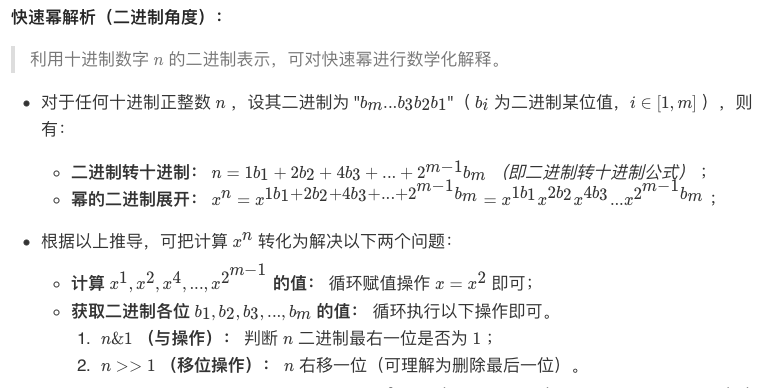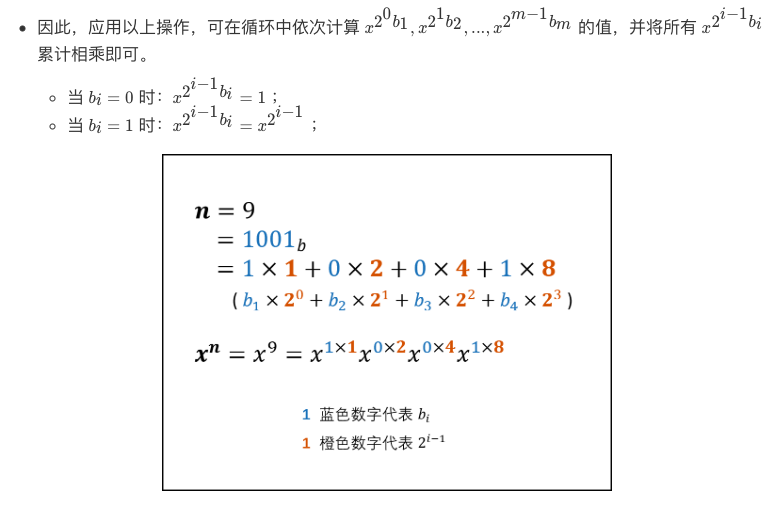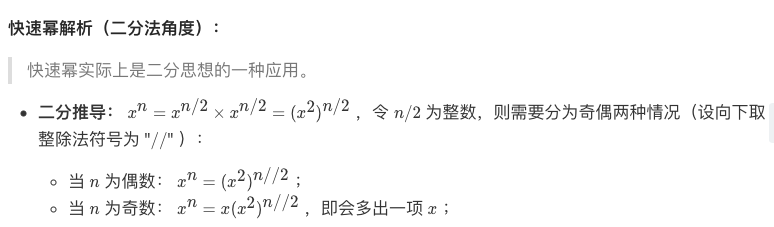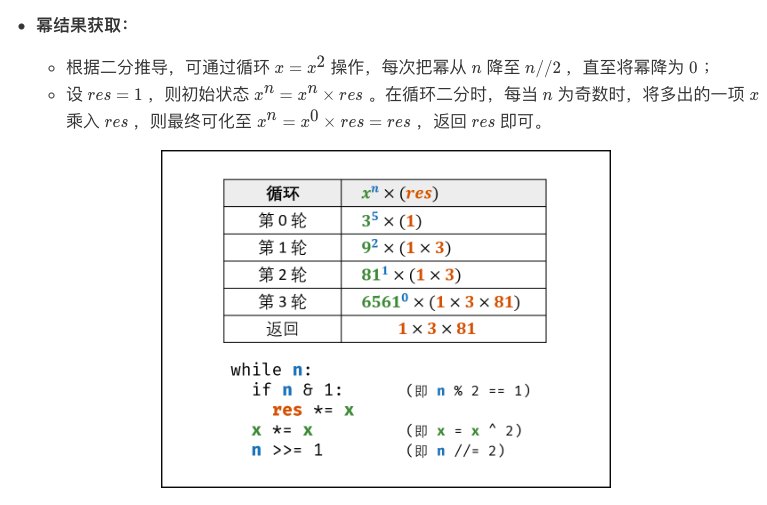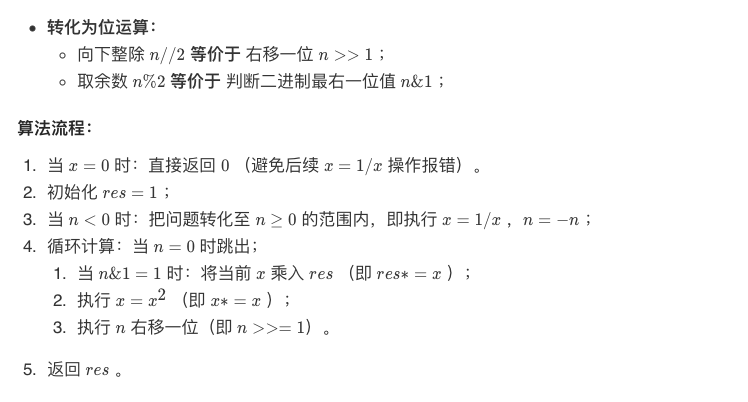int qmi(int m, int k, int p)
{
int res = 1 % p, t = m;
while (k)
{
if (k&1) res = res * t % p;
t = t * t % p;
k >>= 1;
}
return res;
}

import java.io.*;
import java.util.*;

public class Main {
public static void main(String args[]) throws Exception {
Scanner cin=new Scanner(System.in);
/*
res, a 可能会溢出，所以修改为long格式
*/
long a = cin.nextInt();
long b = cin.nextInt();
int p = cin.nextInt();
long res = 1;

while (b > 0) {
if ((b & 1) == 1) {
res *= a;
res %= p;
}
a *= a;
a %= p;
b >>= 1;
}
res %= p;
System.out.println((int)res);
}
}


输入样例：
3
4
5

2


import java.util.*;
public class Main {
public static void main(String[] args) {
Scanner sc = new Scanner(System.in);
long a = sc.nextLong();
long b = sc.nextLong();
long p = sc.nextLong();

System.out.println(a * b % p);
}
}


	long 二进制位数：64
包装类：java.lang.Long
最小值：Long.MIN_VALUE=-9223372036854775808 （-2的63次方）
最大值：Long.MAX_VALUE=9223372036854775807 （2的63次方-1）


int 范围大约为 $-2^{9}$long范围大约为$-2^{18}$，所以直接计算会导致溢出，这就需要我们使用 a + a + a + … + a的方法，因此我们也可以用快速幂思想：

$a * b = a*(b_{0}*2^{0}+b_{1}*2^{1}+...b_{n}*2^{n})$

import java.util.*;
public class Main {
public static void main(String[] args) {
Scanner sc = new Scanner(System.in);
long a = sc.nextLong();
long b = sc.nextLong();
long p = sc.nextLong();
long res = 0;

while (b > 0) {
if ((b & 1) == 1) {
res = (res + a) % p;
}
b >>= 1;
a = (a + a) % p;
}

System.out.println(res);
}
}©️2019 CSDN 皮肤主题: 大白 设计师: CSDN官方博客